# Learn C Language–Tutorial 2: Getting started with C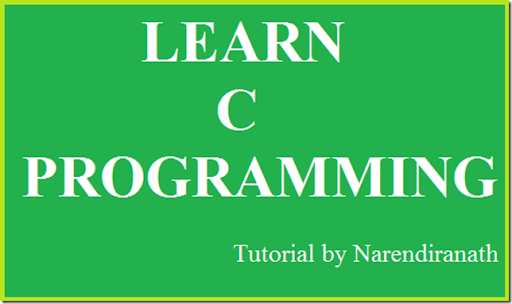Learn C Programming is a series of tutorials on c language. Follow my simple instructions and You also can become the C programmer.
In the first tutorial I have said how to run a C program. You can view the tutorial by clicking the link
http://www.millioninformations.com/2015/08/how-to-run-c-program.html
In that tutorial I have said about What is a C language and the method of how to run the C language. But I have not yet told about the basic things in the C program.
To be tell this in detail consider an example that we learn English language since it is a global language for communication. We do not learn English in a single day, first we learn the alphabets and then we use the alphabets to combine the letters and form a word. Then we learn some grammar to create a sentence. Refer the diagram for clear understanding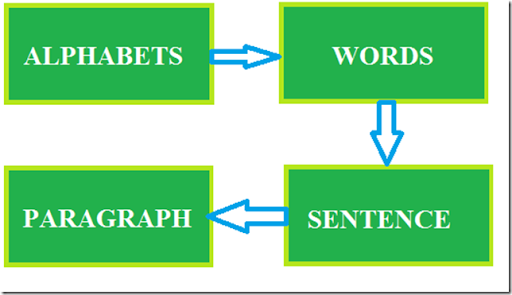Like that in order to learn a C language there are some basic things we need to learn like alphabets, numbers, keywords, special symbols, constants, variables and how these are constructed and used in the C program.
Steps involved in learning C language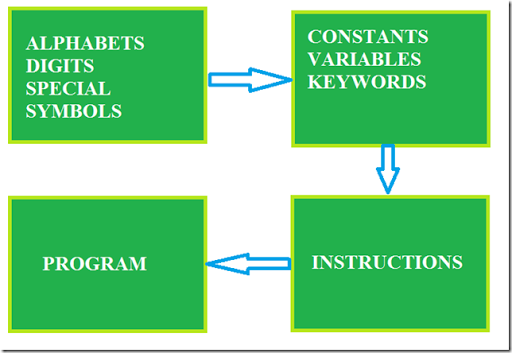In this tutorial i am going to mainly concentrate on the first box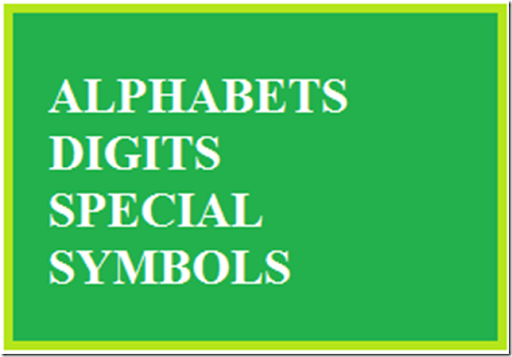Alphabets – A,B……Z and a,b….z
Digits – 0,1,2,3,4,5,6,7,8,9
special symbols – ~!@#\$%^&*()_+=-/?”’>.<,:;[]{}
The alphabets, numbers,and special symbols when properly combined form constants, variables and keywords.
Constants and Variables
A Variable is simple to describe let us assume that to perform a simple addition calculation manually for example
we simply write it in a paper as
5
+
3
----
8
----
So we need a space to write the numbers for calculation for manual calculations we use paper and pen.
So in case of computer calculation how do we do ???
So a storage is required in terms of storing the numbers for calculations. Thus we use alphabets to store the numbers.
we use lower case letters to store a number.
That alphabet is called as a variable and the number stored is called as constants.
In order to use a variable to store some values we need to say to the computer that i am going to use this particular letter to store a value this process is called as variable declaration.
Consider the above example
Consider the below is the storage in computer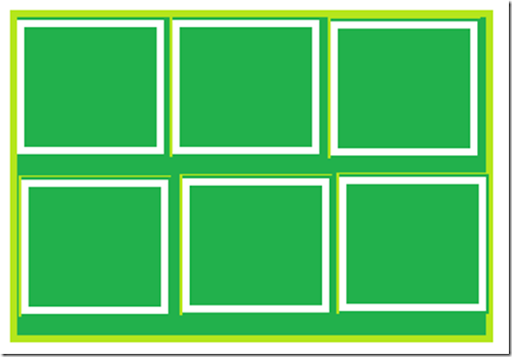If i am going to use a for storing 5 and b for storing 3
and c for storing result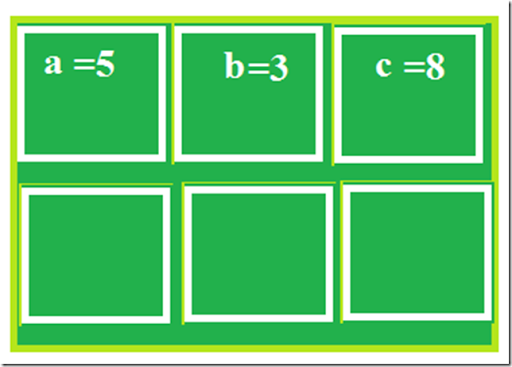Thus the concept of variables and constants are clearly explained.
TYPES OF C CONSTANTS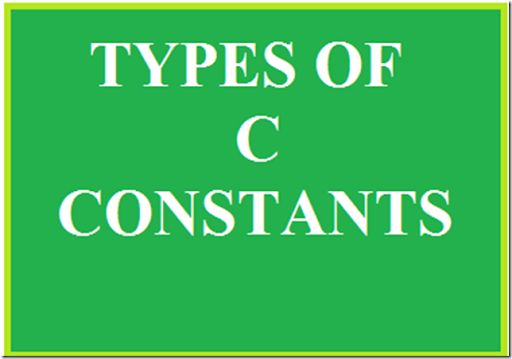There are two types of constants
1.Primary Constants
2.Secondary Constants
Primary Constants includes
• Integer Constants
• Real Constants
• Character Constants
Integer Constants
An Integer Constant must be at least of one digit, it can be a negative or positive the integer constant lies between the range –2147483648n to +2147483647.
ex: 1,2,3000,-250
Real Constant
These are also called as Floating point constants.
A real constant must have at least one digit, it must have a decimal point, default sign is positive but it could have both positive and negative.
ex: +445.566 , –56.45, 456.0
Character Constants
A character constant is a single alphabet written within a inverted commas.
ex: ‘A’ , ‘a’
Types of Variables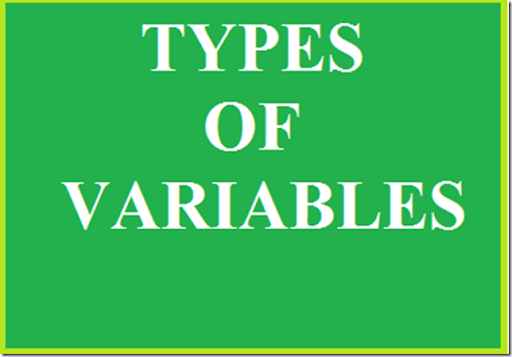The Variable is different for different data types. Data Type is nothing but it is a type of the data that it is a integer or character or String or float.
A variable names are names given to the storage location in memory.
A variable name is any combination of 1 to 31 alphabets, digits,underscores.
The first letter of the variable must be an alphabet or underscore.
No commas or blanks are allowed, no special symbols other than underscore should not be used.
ex: mat_marks, m5_marks, emp_name
If you notice that mat_marks and m5_marks are marks that are numbers and emp_name is a collection of character so how the complier will differentiate between these .
Here the data type is used
The numbers are int types names are char types
decimal values are float types.
ex: int num, char name, float percntage.
Now we are in the final discussion of this tutorial that it is Keyword.
C KEYWORDS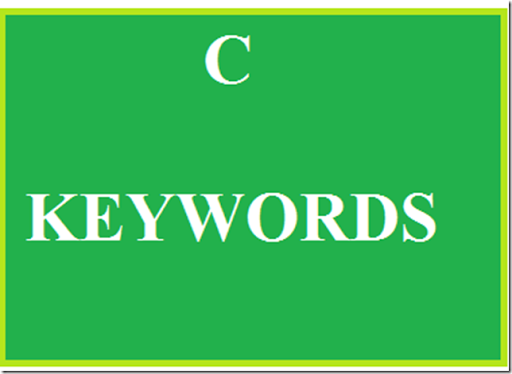Keywords are the predefined words whose meaning is already explained to the C compiler.
The Keywords cannot be used as a variable names.
There are 32 keywords available in C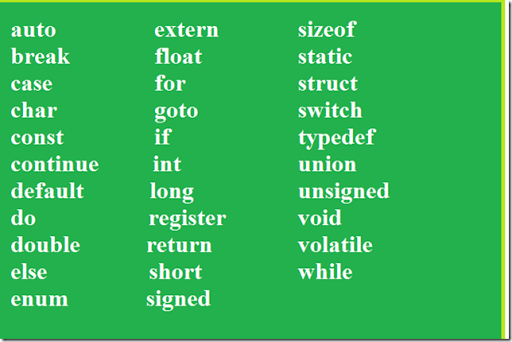In our upcoming tutorials we can deeply see about the above keywords.
I hope this tutorial will thought you some tips in c.
Keep visiting my blog …
stay tuned for further tutorials…